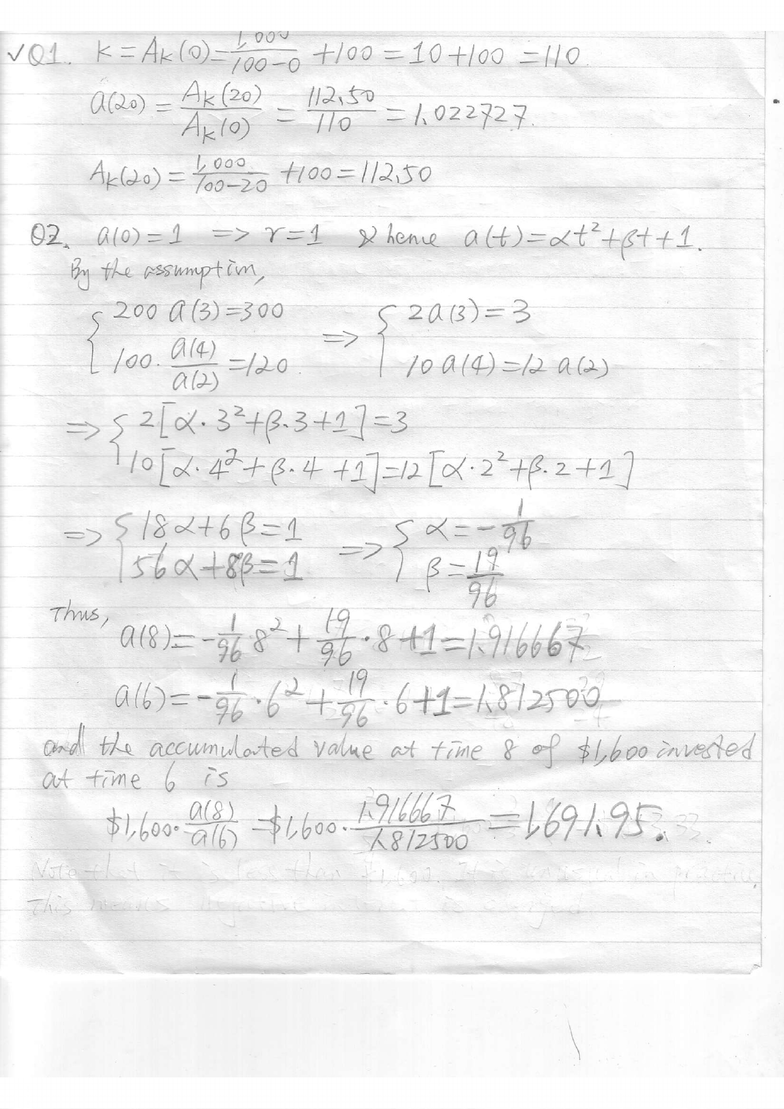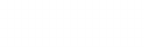Class Notes (1,100,000)
CA (650,000)
UW (20,000)
ACTSC (300)
Lecture

# ASSIGNMENT assignment one

Department
Actuarial Science
Course Code
ACTSC231
Professor
Chengguo Weng

This preview shows pages 1-2. to view the full 7 pages of the document.re-t- 'A/
Afro
--
&@) - //4'eD
-
v\'--
/ - T.@ = llo = l'ozzVzJ
lA- l+1 |
t'zoo
fr(ES-Joo ( 20G):3
( l)trt :) 1
L
/oo
ffir=/Lo I to
a&)
=/t
--\
. .=/
a6)=-f ii-:(? ,
*l=1,,t
ly;pw
, ]b
'o -r:6'o .
b.di tL, aqw Lt!r+e,4 v44ae- 6,t f /l4e V
_&ALlpo ">,Aled
r\f ^\ , t 9l/,
vt\-{)_ Jtt-, l\/(bt,
77t-" -l l/boa '':--'-
-,rg/
I

Only pages 1-2 are available for preview. Some parts have been intentionally blurred.Q3. Label time t= 0 for March 1, 1998. At time 2 (i.e., March 1, 2000), Robin’s balance
is \$6,300(1 + 5%)2= \$6,945.75. After the withdrawal of \$2,000 on March 1, 2000, the
balance becomes \$[6,945.75-2,000]=\$4,945.75.
Let t1be the time length needed for \$4,945,75 to grow to \$5,000 at an annual interest
rate of 3.5%. Then, we have the equation
4.945.75(1 + 3.5%)t1= 5,000.
Solving the above equation, we obtain t1= 0.317117. This implies that the balance
after 2.317117 years (since t=0), is \$5,000. Therefore, on March 1, 2005 (i.e., t=7),
right before the deposit of \$1,000, the balance will be
\$(5,000)(1 + 5%)72.317117 = \$6,283.43.
On March 1, 2010 (t=12), the balance is therefore \$(6,283.43 + 1,000)(1 + 5%)127=
\$9,295.71.